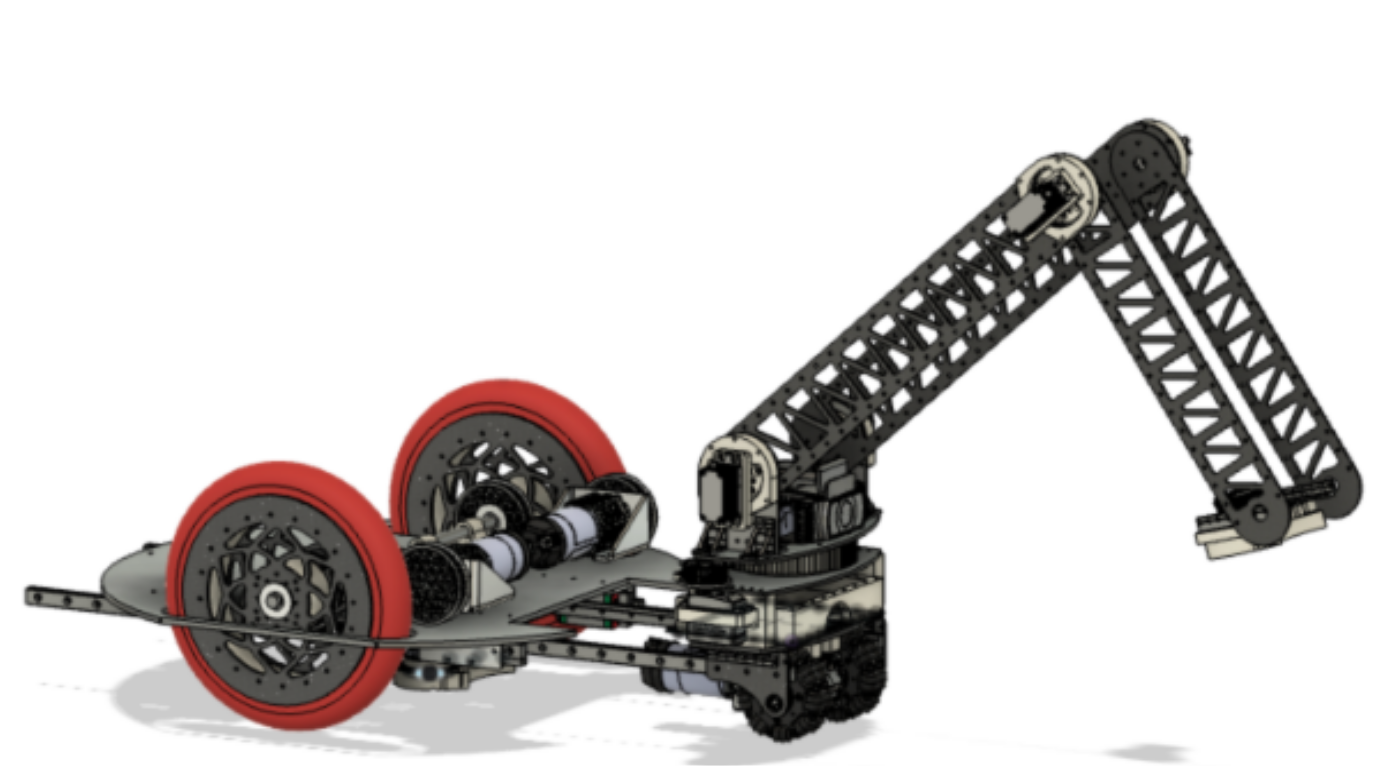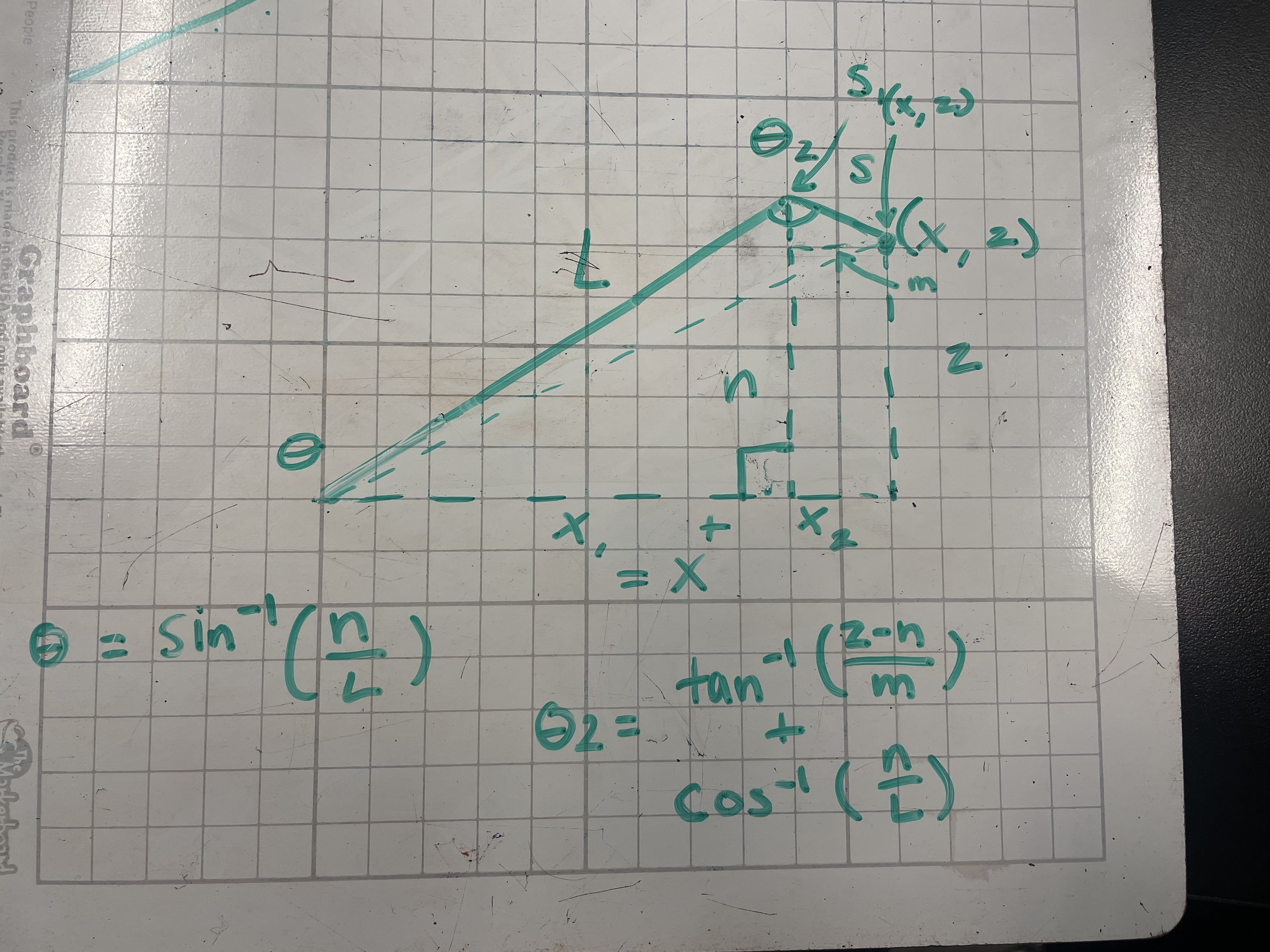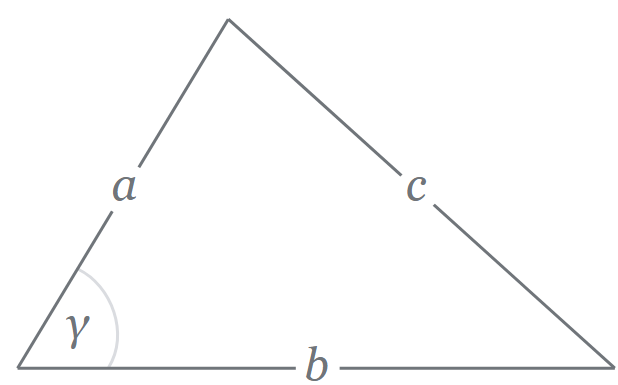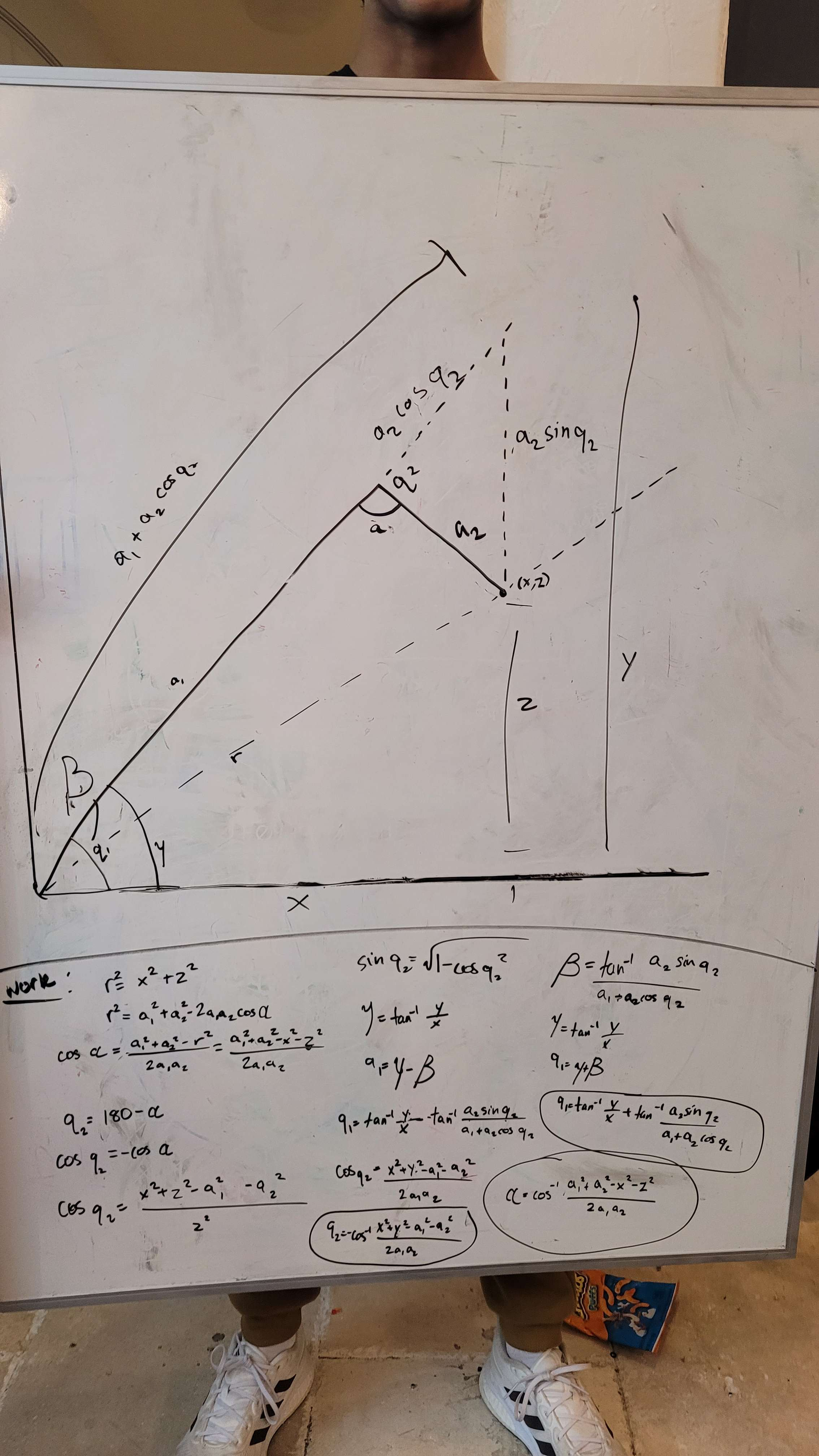# UnderArm Inverse Kinematics

Tags: control, software, and journal
Personhours: 60
UnderArm Inverse Kinematics By Jai, Vance, Alex, Aarav, and Krish

### Task: Implement Inverse Kinematics on the UnderArm

Inverse kinematics is the process of finding the joint variables needed for a kinematic chain to reach a specified endpoint. In the context of TauBot2, inverse kinematics, or IK is used in the crane and the UnderArm. In this blog post, we'll be focused on the implementation of IK in the UnderArm.The goal of this problem was to solve for the angles of the shoulder and the elbow that would get us to a specific field coordinate. Our constants were the end coordinates, the lengths of both segments of our UnderArm, and the starting position of the UnderArm. We already had the turret angle after a simple bearing calculation based on the coordinates of the field target and the UnderArm.

First, we set up the problem with the appropriate triangles and variables. Initially, we tried to solve the problem with solely right angle trigonometry. After spending a couple hours scratching our heads, we determined that the problem wasn't possible with solely right angle trig. Here's what our initial setup looked like.In this image, the target field coordinate is labeled (x, z) and the upper and lower arm lengths are labeled S and L. n is the height of the right triangle formed by the upper arm, a segment of x, and the height of the target. m is the perpendicular line that extends from the target and intersects with n. We solved this problem for θ and θ2, but we solved for them in terms of n and m, which were unsolvable.

After going back to the drawing board, we attempted another set of equations with the law of sines. More frustration ensued, and we ran into another unsolveable problem.

### Our Current Solution

Finally, we attempted the equations one more time, this time implementing the law of cosines. The law of cosines allows us to solve for the side lengths of non-right triangles.Using this, we set up the triangles one more time.Our goals in this problem were to solve for q1 and for α.

We quickly calculated a hypotenuse from the starting point to the target point with the Pythagorean Theorem. Using the radius and our arm length constants we determined cos(α). All we had to do from there was take the inverse cosine of that same equation, and we'd calculated α. To calculate q1, we had to find q2; and add it to the inverse tangent of our full right triangle's height and width. We calculated q2 using the equation at the very bottom of the image, and we had all of the variables that we needed.

After we solved for both angles, we sanity checked our values with Desmos using rough estimates of the arm segment lengths. Here's what the implementation looks like in our robot's code.Date | February 17, 2023